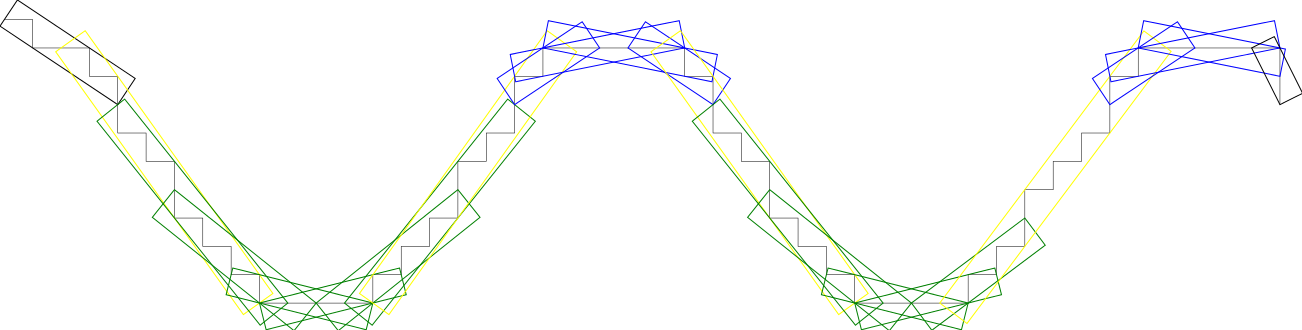DGtal  1.3.beta
geometry/curves/convex-and-concave-parts.cpp

This example outputs the cover of an open digital curve by maximal DSSs. Maximal DSSs are displayed in blue, green, yellow in convex, concave, inflexion parts respectively. Ends are black. Convex (resp. concave) parts are defined as sequences of maximal DSSs of increasing (resp. decreasing) slope.

$./examples/geometry/curves/convex-and-concave-parts  Note that the chain code of the input digital curve may be passed as argument as follows: $ ./examples/geometry/curves/convex-and-concave-parts 0300303303033030303000010101011010110100000303303033030303Decomposition into convex and concave parts
Digital straight lines and segments and Analysis of one-dimensional discrete structures
#include <cmath>
#include <iostream>
#include <sstream>
#include <fstream>
#include "DGtal/base/Common.h"
#include "DGtal/io/boards/Board2D.h"
#include "DGtal/io/Color.h"
#include "DGtal/shapes/Shapes.h"
#include "DGtal/helpers/StdDefs.h"
#include "DGtal/geometry/curves/ArithmeticalDSSComputer.h"
#include "DGtal/geometry/curves/FreemanChain.h"
#include "DGtal/geometry/curves/SaturatedSegmentation.h"
#include "ConfigExamples.h"
using namespace std;
using namespace DGtal;
using namespace Z2i;
template <typename Iterator, typename Board>
void drawCCP(const Iterator& itb, const Iterator& ite, Board& aBoard)
{
//choose the drawing mode
aBoard << SetMode( "ArithmeticalDSS", "BoundingBox" );
//prepare the drawing style and the pen color
string aStyleName = "ArithmeticalDSS/BoundingBox";
CustomPenColor* aPenColor;
//for each maximal segment
for (Iterator i(itb); i != ite; ++i) {
//get the current maximal segment
typedef typename Iterator::SegmentComputer::Primitive DSS;
DSS maximalDSS = i->primitive();
//if located at the end of a connected part
if ( !(i.intersectNext() && i.intersectPrevious()) ) {
aPenColor = new CustomPenColor( Color::Black );
//otherwise
} else {
//get the points located before and after the maximal segment
typedef typename DSS::Point Point;
Point beforeFirst = *(--(i->begin()));
Point afterLast = *(i->end());
//remainders and bounds
typedef typename DSS::Integer Integer;
Integer r1 = maximalDSS.remainder(beforeFirst);
Integer r2 = maximalDSS.remainder(afterLast);
Integer mu = maximalDSS.mu();
Integer omega = maximalDSS.omega();
//configurations
if ( (r1<=mu-1)&&(r2<=mu-1) ) { //concave
aPenColor = new CustomPenColor( Color::Green);
} else if ( (r1>=mu+omega)&&(r2>=mu+omega) ) { //convex
aPenColor = new CustomPenColor( Color::Blue );
} else if ( (r1>=mu+omega)&&(r2<=mu-1) ) { //convex to concave
aPenColor = new CustomPenColor( Color::Yellow );
} else if ( (r1<=mu-1)&&(r2>=mu+omega) ) { //concave to convex
aPenColor = new CustomPenColor( Color::Yellow );
} else { //pb
aPenColor = new CustomPenColor( Color::Red );
}
}
// draw the maximal segment on the board
aBoard << CustomStyle( aStyleName, aPenColor )
<< maximalDSS;
}
}
template <typename Iterator, typename Board>
void segmentationIntoMaximalDSSs(const Iterator& itb, const Iterator& ite,
Board& aBoard)
{
//choose the primitive computer and the segmentation
typedef ArithmeticalDSSComputer<Iterator,Coordinate,4> RecognitionAlgorithm;
//create the segmentation
RecognitionAlgorithm algo;
Segmentation s(itb,ite,algo);
//draw the result
drawCCP(s.begin(), s.end(), aBoard);
}
int main( int argc, char** argv )
{
trace.beginBlock ( "Example convex-and-concave-parts" );
Board2D aBoard; //create a board
//create a chain code
string codes;
if (argc >= 2) codes = argv;
else codes = "030030330303303030300001010101101011010000030330303303030300001010110101011010000033";
stringstream ss(stringstream::in | stringstream::out);
ss << "0 0 " << codes << endl;
FreemanChain<int> theContour( ss );
trace.info() << "Processing of " << ss.str() << endl;
//draw the digital contour
aBoard
<< SetMode( "PointVector", "Grid" )
<< theContour;
//draw the maximal segments
segmentationIntoMaximalDSSs(theContour.begin(), theContour.end(), aBoard);
//save the drawing
aBoard.saveSVG("convex-and-concave-parts.svg");
#ifdef WITH_CAIRO
aBoard.saveCairo("convex-and-concave-parts.png");
#endif
return 0;
}
// //
DGtal::IteratorCirculatorTraits
Aim: Provides nested types for both iterators and circulators: Type, Category, Value,...
Definition: IteratorCirculatorTraits.h:294
DGtal::Trace::endBlock
double endBlock()
DGtal::ArithmeticalDSSComputer
Aim: This class is a wrapper around ArithmeticalDSS that is devoted to the dynamic recognition of dig...
Definition: ArithmeticalDSSComputer.h:86
LibBoard::Board::saveCairo
void saveCairo(const char *filename, CairoType type=CairoPNG, PageSize size=Board::BoundingBox, double margin=10.0) const
Definition: Board.cpp:1139
DGtal::trace
Trace trace
Definition: Common.h:154
DGtal::FreemanChain< int >
DGtal::Trace::beginBlock
void beginBlock(const std::string &keyword="")
DGtal::CustomStyle
Definition: Board2D.h:217
DGtal::Trace::info
std::ostream & info()
DGtal
DGtal is the top-level namespace which contains all DGtal functions and types.
LibBoard::Board::saveSVG
void saveSVG(const char *filename, PageSize size=Board::BoundingBox, double margin=10.0) const
Definition: Board.cpp:1012
drawCCP
void drawCCP(const Iterator &itb, const Iterator &ite, Board &aBoard)
Definition: testSegmentation.cpp:93
Segmentation
SaturatedSegmentation< SegmentComputer > Segmentation
Definition: testArithmeticalDSSComputerOnSurfels.cpp:56
main
int main(int argc, char **argv)
Definition: testArithmeticDSS-benchmark.cpp:147
Integer
Point::Coordinate Integer
DGtal::Board2D
Aim: This class specializes a 'Board' class so as to display DGtal objects more naturally (with <<)....
Definition: Board2D.h:70
DGtal::SetMode
Modifier class in a Board2D stream. Useful to choose your own mode for a given class....
Definition: Board2D.h:247
segmentationIntoMaximalDSSs
void segmentationIntoMaximalDSSs(const Iterator &itb, const Iterator &ite, const Iterator &sitb, const Iterator &site, const string &aMode, Board &aBoard)
Definition: testSegmentation.cpp:175
DGtal::SaturatedSegmentation
Aim: Computes the saturated segmentation, that is the whole set of maximal segments within a range gi...
Definition: SaturatedSegmentation.h:153
Point
MyPointD Point
Definition: testClone2.cpp:383
DGtal::CustomPenColor
Custom style class redefining the pen color. You may use Board2D::Color::None for transparent color.
Definition: Board2D.h:312